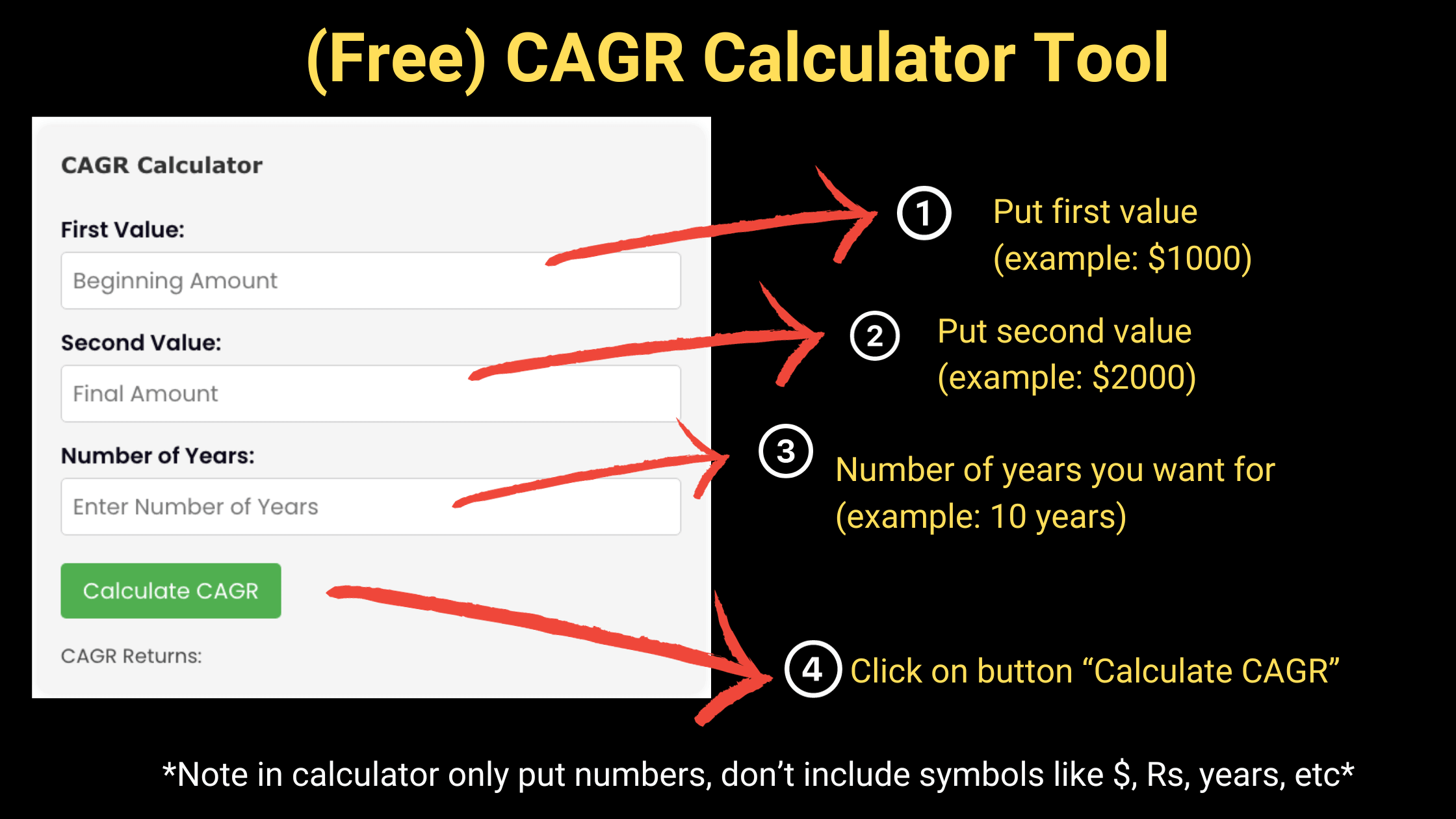cagr calculator online

## CAGR Calculator Online Tool: Free CAGR Return Calculator

CAGR Calculator Online Tool: Set First & Second Value > Set Years > Calculate CAGR

## CAGR Calculator

CAGR Returns:

Investing is a journey, and understanding the growth of your investments is crucial for making informed decisions. One powerful tool that can aid in this understanding is the CAGR calculator online.

CAGR, or Compound Annual Growth Rate, is a term you might have come across, but do you know how vital it is for assessing the performance of your investments over time?

In this blog, we delve deep into the world of CAGR, its significance, and how you can utilize the CAGR calculator online to evaluate your investment growth.

## Understanding CAGR

Before we jump into the functionalities of the CAGR calculator online, let’s first understand what CAGR is. CAGR stands for Compound Annual Growth Rate, and it is a measure of the mean annual growth rate of an investment over a specified time period longer than one year.

It represents one of the most accurate ways to calculate and determine returns for anything that can rise or fall in value over time.

## Why Choose CAGR Calculator Online?

Investors and financial analysts use CAGR to understand and compare the past performance of one investment with other investments in a portfolio, or to project their expected future returns.

With the “cagr return calculator”, “cagr calculator”, and “compound annual growth rate calculator” at your disposal, analyzing your investment’s performance has never been easier.

## CAGR Calculator: An Indispensable Tool for Investors

Now, let’s focus on our main topic of discussion – the CAGR calculator online.

### What is this Tool?

The CAGR Calculator is a simple yet powerful tool that calculates the Compound Annual Growth Rate (CAGR) of an investment. It is designed to provide you with a clear picture of your investment’s growth over a certain period.

### How Does it Work?

The calculator operates using a straightforward formula:
[ CAGR = \left( \frac{Final Amount}{Beginning Amount} \right)^\frac{1}{Number of Years} – 1 ]
This formula helps in determining the geometric progression ratio that yields a constant rate of return over a specified time period.

### How to Use the CAGR Calculator?

Utilizing the CAGR calculator online is a breeze. Here’s a step-by-step guide:

1. Input the Beginning Amount (the initial value of your investment).
2. Input the Final Amount (the value of your investment at the end of the period).
3. Specify the Number of Years over which the growth has occurred.
4. Click on the “Calculate CAGR” button.
5. The calculator will promptly display the CAGR value as a percentage.

### Example:

Let’s run through an example for a clearer understanding:

1. Beginning Amount: $1,000 2. Final Amount:$2,000
3. Number of Years: 10
4. After clicking “Calculate CAGR”, you’ll find that the result is approximately 7.18%. This indicates that the investment has grown at an average rate of 7.18% annually over the 10 years.

## Benefits of Using CAGR Calculator Online

The “sip cagr calculator” and other variations of the CAGR calculator are invaluable tools for any investor looking to assess the performance of their investments. They offer precision, ease of use, and quick results.

By understanding how your investments have performed in the past, you can make more informed decisions for the future.

## Conclusion

Investing smartly requires the right tools and a solid understanding of how to use them. The CAGR calculator online is one such tool that every investor should have in their arsenal.

Whether you are evaluating the performance of stocks, bonds, or any other type of investment, understanding the Compound Annual Growth Rate is crucial. So, go ahead, give our CAGR calculator a try, and take the first step towards smarter, more informed investment decisions. Happy investing!